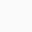# Molarity of 37% (w/w) Hydrochloric Acid (HCl)

## OVERVIEW

• A 37% (w/w) Hydrochloric Acid is a clear colorless aqueous solution of Hydrogen chloride (HCl) gas. It can be purchased from many commercial suppliers.
• % refers to solution concentration in percentage and “(w/w)” refers to solute and solution amount given in grams (i.e., percentage by weight). This means a 37% (w/w) Hydrochloric acid contains 37 g of HCl per 100 g of solution.
• The density of 37% (w/w) Hydrochloric acid solution is 1.2 g/ml at 25° C. This means the weight of the 1 ml of hydrochloric acid solution is 1.2 g at 25°C.
• Molarity refers to the number of moles of the solute present in 1 litre of solution. To calculate the Molarity of 37% (w/w) Hydrochloric Acid (HCl), you need to know how many moles of HCl are present in 1 liter of solution.
• In simple words, 1 mole is equal to the molecular weight of the substance. For example, 1 mole of Hydrogen chloride is equal to 36.46 g of hydrogen chloride (Molecular Weight: 36.46).
• To calculate the molarity, one must first calculate how much Hydrogen chloride is present in 1 L of 37% Hydrochloric acid. Once we know the amount of HCl present in 1 L solution, we can calculate the molarity of the solution by dividing the HCl amount by the molecular weight.
• Calculator: Converting Percentage by Mass to Molarity# Calculation procedure

## Step 1: Calculate the volume of 100 grams of Hydrochloric acid.Formula:

Density = $\frac{Weight}{Volume}$

OR

Volume = $\frac{\mathrm{Weight}}{\mathrm{Density}}$

The volume of 100 g of Hydrochloric acid : $\frac{100}{1.2}$ = 83.33 ml

Note: 37% (w/w) Hydrochloric acid means that 100 g of Hydrochloric acid contains 37 g of HCl.

The volume of 100 g of Hydrochloric acid is 83.33 ml which means 37 g of HCl is present in 83.33 ml of Hydrochloric acid.

## Step 2: Calculate how many grams of HCl is present in 1000 ml of Hydrochloric acid.

83.33 ml of Hydrochloric acid contains   = 37 grams of HCl
1 ml of Hydrochloric acid will contain      =   $\frac{37}{83.33}$ grams of HCl

1000 ml of Hydrochloric acid will contain =   = 444.01776 grams of HCl

1000 ml of Hydrochloric acid will contain 444.01776 grams of HCl.

## Step 3: Calculate the number of moles of HCl in 444.01776 grams of HCl.

36.46 g of HCl is equal to 1 mole.

1 g of HCl will be equal to $\frac{1}{36.46}$ moles.

444.01776 grams of Hydrogen chloride will be equal to = = 12.178 moles

Therefore, we can say that 1 liter of Hydrochloric acid contains 12.178 moles of HCl or in other words molarity of 37% (w/w) Hydrochloric acid is equal to 12.178 M.

# Calculator – Calculate the molarity of concentrated Hydrochloric acid (HCl)

Use Calculator to calculate the molarity of concentrated Hydrochloric acid (HCl) when concentration is given in % by mass (w/w)

Hydrogen Chloride (HCl) Molecular weight: 36.46 g/mole

Concentration of Hydrochloric acid: % (w/w)
(Change the % (w/w) concentration)

Density of Hydrochloric acid: g/ml
(Change the density)

Molarity of Hydrochloric acid: 12.178 M## 9 thoughts on “Molarity of 37% (w/w) Hydrochloric Acid (HCl)”

1.b says:

I think the calculation above is wrong. I think the proper answer is as follows:
37w/w% means that of 1000g of solution 370g is HCl. 370g / 36.46g = 10.15M

2.barry says:

ok wait now its clear to me:
1L of 37w/w% HCl weighs 1200g
37w% of 1200g is 444g
444g / 36.5g = 12.2M